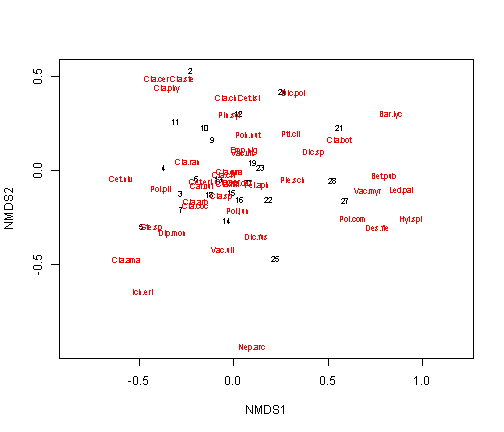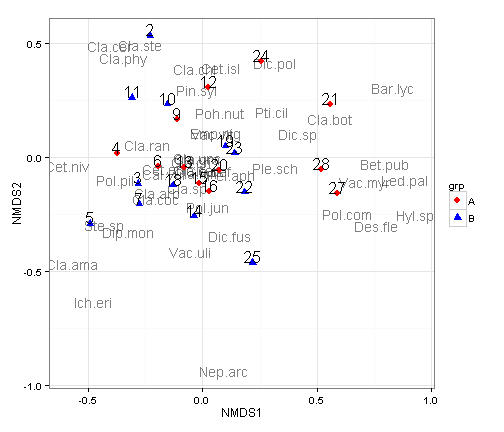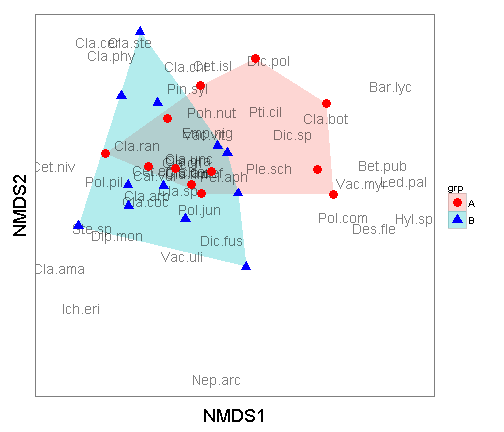# Plotting NMDS plots with ggplot2

The RMarkdown source to this file can be found here

One of my favorite packages in R is ggplot2, created by Hadley Wickham. This package allows you to create scientific quality figures of everything from shapefiles to NMDS plots. I will run through a walkthrough in how to make a NMDS plot using the vegan package and ggplot2. I am not going to go into the details into running the NMDS, as for this walkthrough I am making the assumption you already have a finalized output.

### Load the libraries and get the data

For this walkthrough I would like to assign a “group” to the each row of the data for illustration purposes. Normally, your data will already belong to a grp and this next step will not be necessary.

The basic process I will use to assign these groups is to to find the number of rows of the varespec data and then randomly sample half rows to group ‘A’ and the other half will be group ‘B’.

### Run the NMDS using the vegan package

I am not a fan of using base R for graphics. When you are in a pinch, they are ok to call but never hand in an assignment or attempt to submit for a publication the default plots.### Using ggplot for the NMDS plot

The first step is to extract the scores (the x and y coordinates of the site (rows) and species and add the grp variable we created before. Once again the grp variable is not needed, I am just using it for illustration purposes. For the data.scores, the result will be a 26 row x 4 column data.frame with the NMDS1 (x location) and NMDS2 (y location), designated by the site number and the group (grp). The species.scores will be a 44 row by 3 column data.frame with the NMDS1 (x location), NMDS2 (y location), and species.

Now that we have the site and species scores, we can begin plotting with ggplot2. First we will produce a plot like the base plot function.There are a couple of changes I like to make in the themes to make these a little nicer.Another way to look at these is to plot a hull around each of the groups. To accomplish this, you can utilize the chull function. In the below plot I dropped the site score labels.

and plot it out There are a couple of changes I like to make in the themes to make these a little nicer.ggplot2 gives you a lot of flexibility in developing plots. Whenever you are thinking of plotting with ggplot2 you need to first get the data in a data.frame format. Additionally, because ggplot2 is based on the “Grammar of Graphics” by Leland Wilkinson, you can only have two-axis. Given that, each layer must have the same x and y colummn names. In addition, the plots are built in layers. If in the above plot, if you were to put the geom_polygon below the geom_point line then the hulls would cover up the points and text.

Tags:

Categories:

Updated: